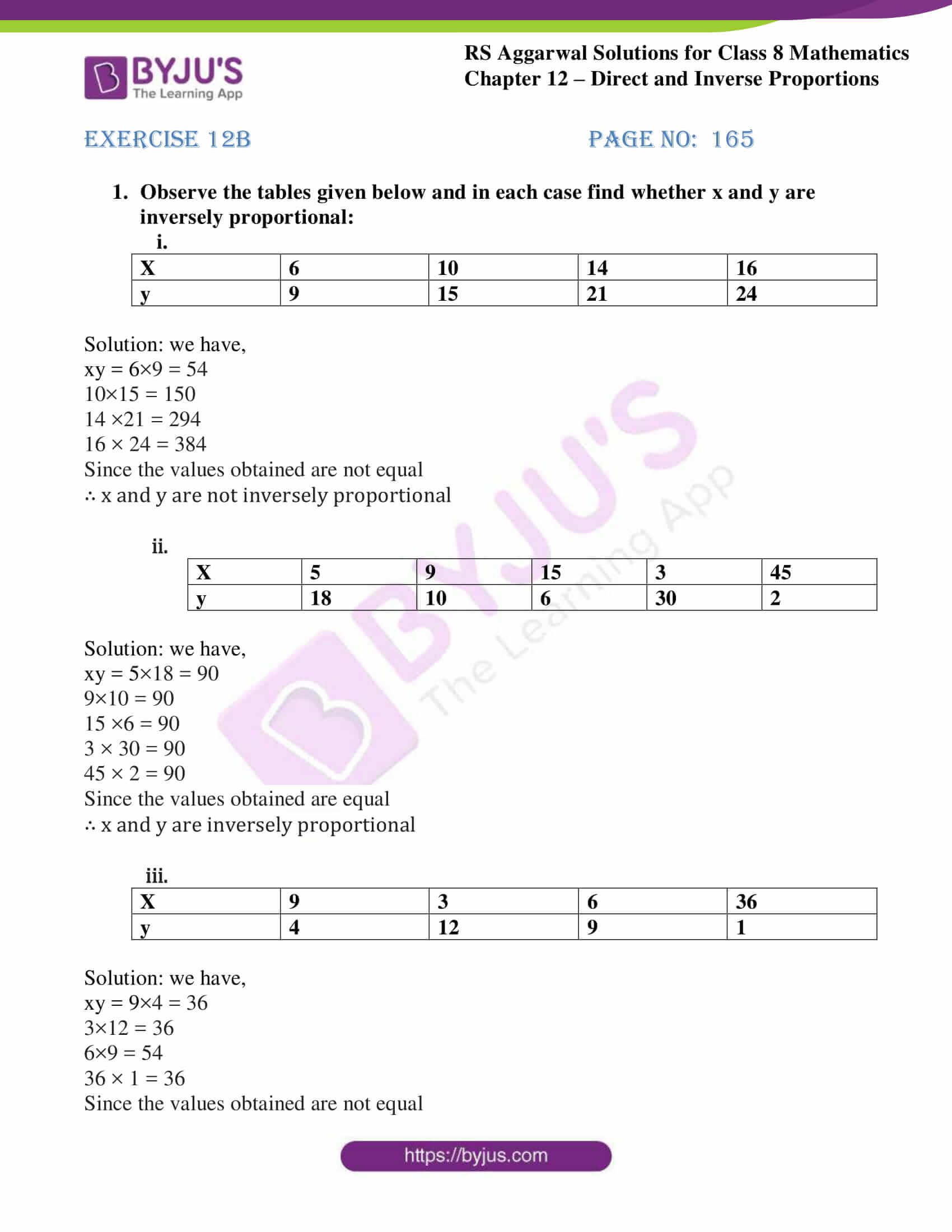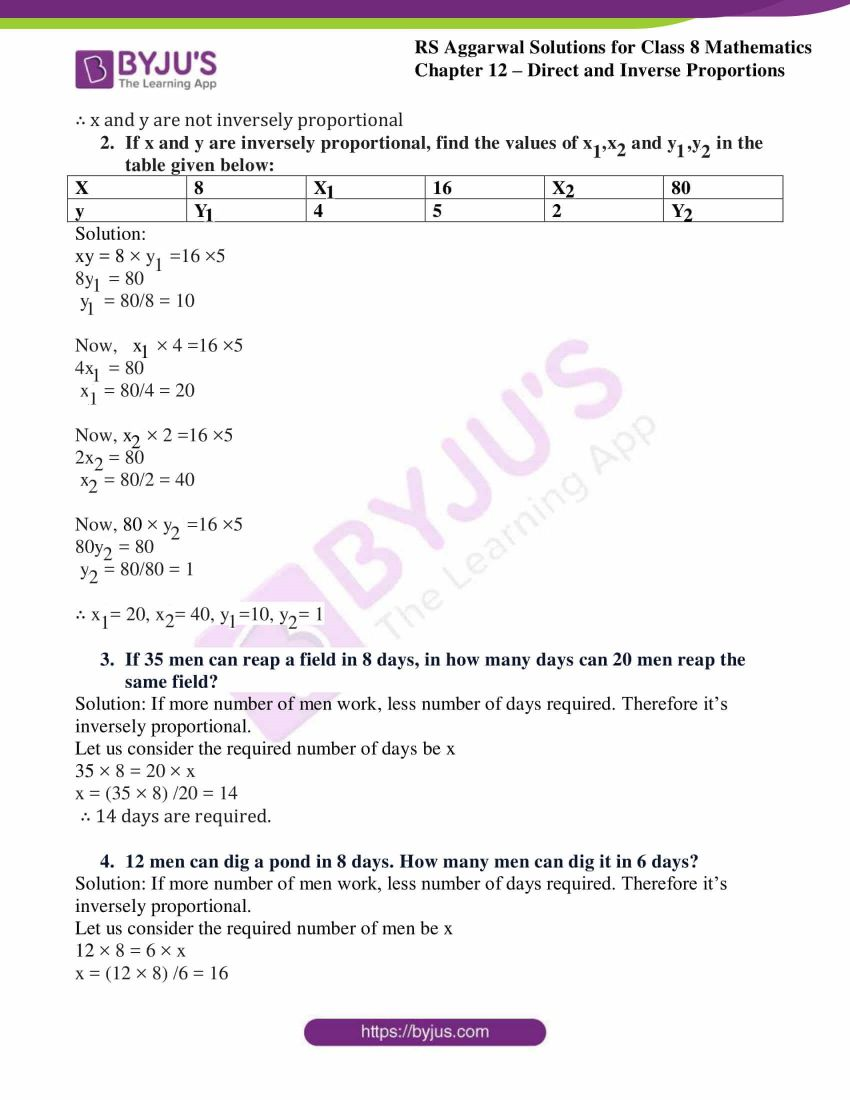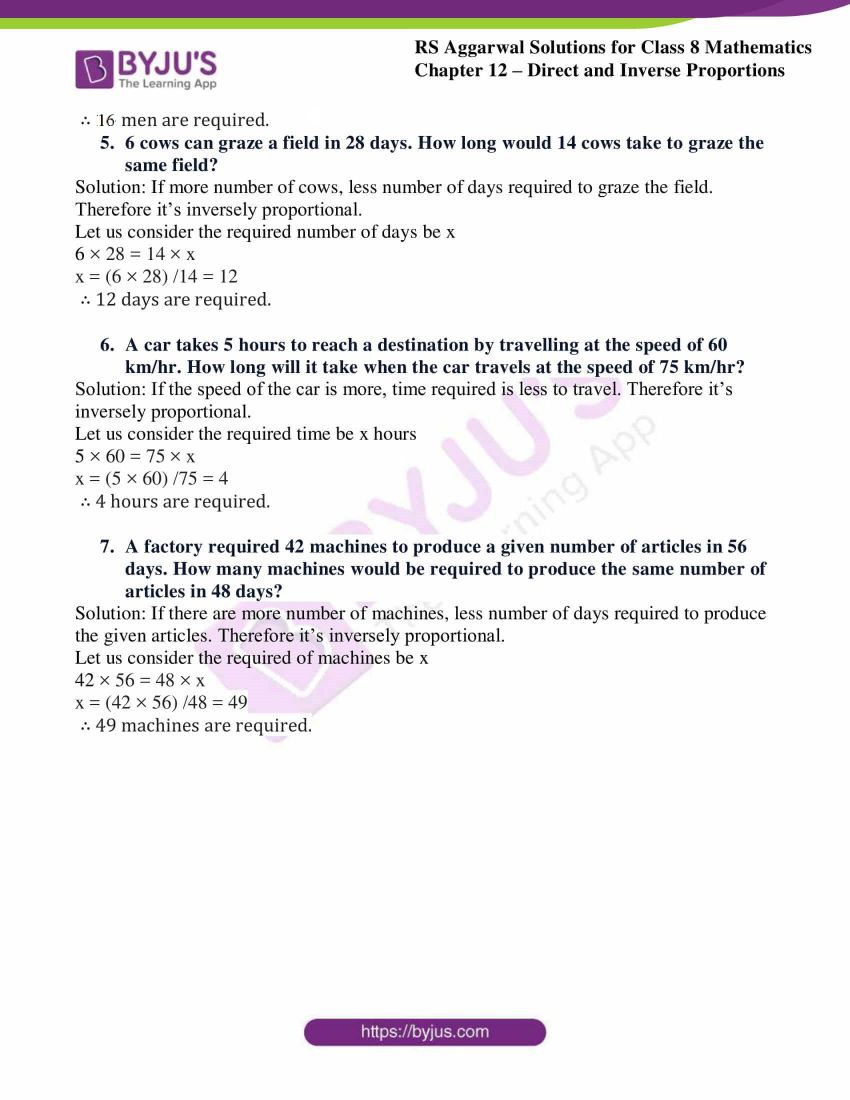# RS Aggarwal Solutions for Class 8 Maths Chapter 12 - Direct and Inverse Proportions Exercise 12B

Download RS Aggarwal Solutions for Class 8 Maths Chapter 12- Exercise 12B, Direct and Inverse Proportions from the links provided below. Our experts have solved the RS Aggarwal Solutions to ensure that the students are thorough with their fundamentals by practicing the solutions. In Exercise 12B of RS Aggarwal Class 8 Maths, we shall see Inverse Proportion.

## Download PDF of RS Aggarwal Solutions for Class 8 Maths Chapter 12- Direct and Inverse Proportions Exercise 12B### Access Answers to RS Aggarwal Solutions for Class 8 Maths Chapter 12- Direct and Inverse Proportions Exercise 12B

1. Observe the tables given below and in each case find whether x and y are inversely proportional:
(i).

 X 6 10 14 16 y 9 15 21 24

Solution: we have,

xy = 6×9 = 54

10×15 = 150

14 ×21 = 294

16 × 24 = 384

Since the values obtained are not equal

∴ x and y are not inversely proportional

(ii).

 X 5 9 15 3 45 y 18 10 6 30 2

Solution: we have,

xy = 5×18 = 90

9×10 = 90

15 ×6 = 90

3 × 30 = 90

45 × 2 = 90

Since the values obtained are equal

∴ x and y are inversely proportional

(iii).

 X 9 3 6 36 y 4 12 9 1

Solution: we have,

xy = 9×4 = 36

3×12 = 36

6×9 = 54

36 × 1 = 36

Since the values obtained are not equal

∴ x and y are not inversely proportional

2. If x and y are inversely proportional, find the values of x1,x2 and y1,y2 in the table given below:

 x 8 x1 16 x2 80 y y1 4 5 2 y2

Solution:

xy = 8 × y1 =16 ×5

8y1 = 80

y1 = 80/8 = 10

Now, x1 × 4 =16 ×5

4x1 = 80

x1 = 80/4 = 20

Now, x2 × 2 =16 ×5

2x2 = 80

x2 = 80/2 = 40

Now, 80 × y2 =16 ×5

80y2 = 80

y2 = 80/80 = 1

∴ x1= 20, x2= 40, y1=10, y2= 1

3. If 35 men can reap a field in 8 days, in how many days can 20 men reap the same field?

Solution: If more number of men work, less number of days required. Therefore it’s inversely proportional.

Let us consider the required number of days be x

35 × 8 = 20 × x

x = (35 × 8) /20 = 14

∴ 14 days are required.

4. 12 men can dig a pond in 8 days. How many men can dig it in 6 days?

Solution: If more number of men work, less number of days required. Therefore it’s inversely proportional.

Let us consider the required number of men be x

12 × 8 = 6 × x

x = (12 × 8) /6 = 16

∴ 16 men are required.

5. 6 cows can graze a field in 28 days. How long would 14 cows take to graze the same field?

Solution: If more number of cows, less number of days required to graze the field. Therefore it’s inversely proportional.

Let us consider the required number of days be x

6 × 28 = 14 × x

x = (6 × 28) /14 = 12

∴ 12 days are required.

6. A car takes 5 hours to reach a destination by travelling at the speed of 60 km/hr. How long will it take when the car travels at the speed of 75 km/hr?

Solution: If the speed of the car is more, time required is less to travel. Therefore it’s inversely proportional.

Let us consider the required time be x hours

5 × 60 = 75 × x

x = (5 × 60) /75 = 4

∴ 4 hours are required.

7. A factory required 42 machines to produce a given number of articles in 56 days. How many machines would be required to produce the same number of articles in 48 days?

Solution: If there are more number of machines, less number of days required to produce the given articles. Therefore it’s inversely proportional.

Let us consider the required of machines be x

42 × 56 = 48 × x

x = (42 × 56) /48 = 49

∴ 49 machines are required.

### Access other Exercises of RS Aggarwal Solutions for Class 8 Maths Chapter 12- Direct and Inverse Proportions

Exercise 12A Solutions 15 Questions

Exercise 12C Solutions 10 Questions

## RS Aggarwal Solutions for Class 8 Maths Chapter 12- Direct and Inverse Proportions Exercise 12B

Exercise 12B of RS Aggarwal Class 8, Direct and Inverse Proportions. This exercise mainly deals with Inverse Proportion- Two quantities x and y are said to be in inverse proportion if xy=k, where k is a constant.

Students are suggested to try solving the questions from RS Aggarwal book of Class 8 and then refer to these solutions to know the best way of approaching the different questions. By referring to these solutions, students develop problem-solving abilities and can tackle any type of questions easily. Thus practicing as many times as possible helps in building time management skills and also boosts the confidence level to achieve high marks.